## Tip: 2 Ways to Create Optimized Score Code with Custom Transformations Generated in a SAS Code Node

Started ‎07-23-2014 by
Modified ‎10-06-2015 by
Views 1,934

Many users turn to the SAS Code node to create custom transformations that will be used in creating a model. Once that model is built, how do you ensure that only the variables used in that model, including custom transformations, will be incorporated in the optimized scoring code? For example, if you're creating new variables X1 and X2, but X2 is the only variable in the final model, you do not want the creation of X1 to appear in your optimized scoring code.

The SAS® Enterprise Miner™ environment does not parse score code, so in order to assemble the necessary pieces for optimized score code, new variables must be registered in a table with the corresponding formula or mapped to separate scoring files. In either case, the EMSCOREVAR dataset must be created to house this information. This table must contain the following variables:

• NAME: name of the new variable created in your scoring code
• FORMULA: string containing the formula to create the new variable
• FILE: name of the file containing scoring code to create the new variable

For transformations that are easily mapped to simple formulas, using the FORMULA field in the EMSCOREVAR table is recommended; for more complex transformations, using the FILE field to create individual score files is a better approach. Let’s walk through an example of each scenario.

Example 1: Simple Transformations

Using sampsio.hmeq (with BAD=TARGET), I want to create two simple LOG transformations within the SAS Code Node to be used as candidates in building a Decision Tree.  Once this tree is built, I want my corresponding optimized scoring code to contain information only on those variables used in building my model. I create the following flow in Enterprise Miner.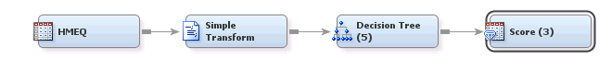I open the SAS Code Node (labeled Simple Transform) and enter the following code in the Score Code section of my code editor to create two new variables that are LOG transformations of existing variables in my training data. This will be used to build the EMFLOWSCORE.sas and/or EMPUBLISHSCORE.sas files that will be used in the accumulated path scoring code.

log_loan = log(loan)

log_mortdue = log(mortdue);

I then toggle to the Training Code section of my code editor and begin the work necessary to build the optimized scoring code for this task. In this example, I simply need to build the EMSCOREVAR table and include an entry for each of these new variables as well as the simple formulas associated with them. This is done using the following code:

/* create EMSCOREVAR table that map new variables to associated score files *

%em_register(key=EMSCOREVAR, type=DATA);

%let scorevarDs =%scan(&em_user_emscorevar, 2, .);

proc datasets lib=&em_lib nolist;

delete &scorevarDs;

run;

quit;

/* assigning formulas to the new variables */

data &em_user_emscorevar;

length Name \$32 formula \$70 file \$200;

keep NAME Formula file;

NAME="log_mortdue";

Formula="log(mortdue)";

file="";

output;

NAME="log_loan";

Formula="log(loan)";

file="";

output;

run;

After the SAS Code node has run, I can verify that the EMCODE_EMSCOREVAR table exists in the EMWS folder, with an entry for both new variables defining the transformation definition.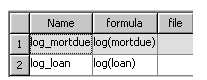I next view the variables passed into the Decision Tree node and verify that log_mortdue and log_loan have both been created by the EMFLOWSCORECODE.sas file from my SAS Code node, and are available as splitting variables in the Decision Tree node. At this point, I open Interactive Training for the Decision Tree Node and force a split on the root node using my new variable log_loan. Now I save this change by closing interactive training. This will create a Decision Tree model in which only the log_loan variable is needed for scoring. I now run the Score Node and view the results of this node when the run completes.

You can see that the SAS Code displayed in the results of this node contains scoring code for ALL of the new transformations I created in the SAS Code node. in addition to the scoring code for the Decision Tree model. See snippet of code below: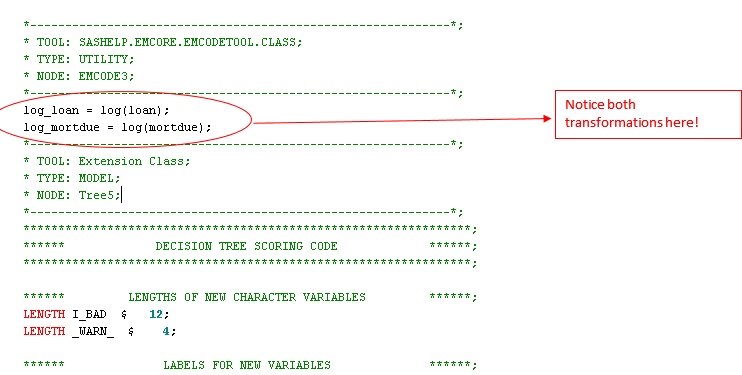However, if I look to the Optimized SAS Code displayed in these results, you see that only log_loan is included in the scoring code in the EMCODETOOL section of scoring code. This is because this is the only variable that was actually necessary for building the Decision Tree. There is no need for the optimized scoring code to create log_mortdue.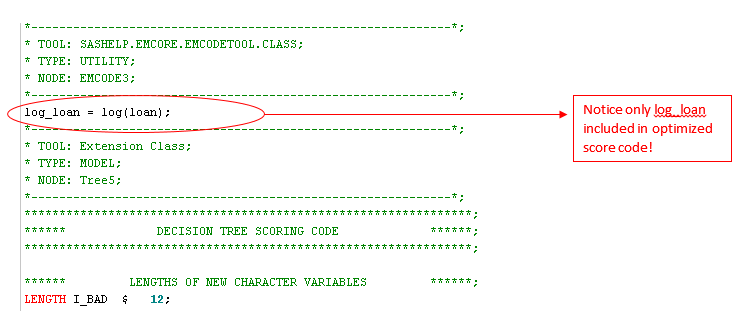Example 2: More Complex Transformations

Using sampsio.hmeq (with BAD=TARGET), I want to create more complex transformations in which I create group variables based on cutoff values of original variables in my training table. This is too complicated to define in the Formula column of the EMSCOREVARS table as we did in Example 1. This example will demonstrate how a user can pass in a FILE value for the transformation definition. This transformation will then be used as a candidate in building a Decision Tree as was done in Example 1. Once this tree is built, I want my corresponding optimized scoring
code to  contain information only on those variables used in building my model. I create the following flow in Enterprise Miner: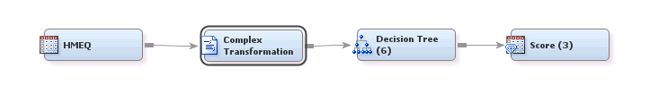In this example, I submit the following in the Score Code of the SAS Code node to create two new variables, GRP_CLAGE and GRP_CLNO. These variables will have 5 values each and will be created based on cutoff values in my original CLAGE and CLNO variables.

*------------------------------------------------------------*;

* Variable: CLAGE;

*------------------------------------------------------------*;

LABEL GRP_CLAGE = "Grouped: CLAGE";

if MISSING(CLAGE) then do;

GRP_CLAGE = 1;

end;

else if NOT MISSING(CLAGE) then do;

if CLAGE < 115.1 then do;

GRP_CLAGE = 2;

end;

else if 115.1 <= CLAGE AND CLAGE <173.47 then do;

GRP_CLAGE = 3;

end;

else if 173.47 <= CLAGE AND CLAGE <231.57 then do;

GRP_CLAGE = 4;

end;

else if 231.57 <= CLAGE then do;

GRP_CLAGE = 5;

end;

end;

*------------------------------------------------------------*;

* Variable: CLNO;

*------------------------------------------------------------*;

LABEL GRP_CLNO = "Grouped: CLNO";

if MISSING(CLNO) then do;

GRP_CLNO = 1;

end;

else if NOT MISSING(CLNO) then do;

if CLNO < 15 then do;

GRP_CLNO = 2;

end;

else if 15 <= CLNO AND CLNO < 20 then do;

GRP_CLNO = 3;

end;

else if 20 <= CLNO AND CLNO < 26 then do;

GRP_CLNO = 4;

end;

else if 26 <= CLNO then do;

GRP_CLNO = 5;

end;

end;

You can quickly see why it would be too complicated to include these formulas in the Formula cell of the EMSCOREVAR table. Instead, I first create a new subfolder (BINCODE) under the node folder and then create an individual score file within BINCODE for each new transformation variable. I then provide a mapping to these files in the EMSCOREVAR table. The following code can be run within the Train Code of the SAS Code node to do this.

/* create EMSCOREVAR table that maps new variables to associated score files */

%em_register(key=EMSCOREVAR, type=DATA);

%let scorevarDs = %scan(&em_user_emscorevar, 2, .);

proc datasets lib=&em_lib nolist;

delete &scorevarDs;

run;

quit;

/* Retrieve the name of subfolder in your node folder */

%em_getName(key=BINCODE, type=FOLDER);

/* generate individual files to support optimized score code for more complicated transformations */

/* Create the subfolder */

data _null_;

length rc \$200;

rc = dcreate("BINCODE", "&EM_NODEDIR");

run;

/* Create the _ALL_.sas file */

filename _F1 "&em_user_bincode&em_dsep._ALL_.sas";

data _null_;

file _F1;

put 'length _UFormat \$200;';

put 'drop _UFormat;';

put "_UFormat=''; ";

run;

filename F1;

/* Create a file for GRP_CLAGE transformation in the subfolder */

filename _F1 "&em_user_bincode&em_dsep.GRP_CLAGE.sas";

data _null_;

file _F1;

put 'if MISSING(CLAGE) then do;';

put '  GRP_CLAGE = 1;';

put 'end;';

put 'else if NOT MISSING(CLAGE) then do;';

put '  if CLAGE < 115.1 then do;';

put '    GRP_CLAGE = 2;';

put '  end;';

put '  else if 115.1 <= CLAGE AND CLAGE < 173.47 then do;';

put '    GRP_CLAGE = 3;';

put '  end;';

put '  else if 173.47 <= CLAGE AND CLAGE < 231.57 then do;';

put '    GRP_CLAGE = 4;';

put '  end;';

put '  else if 231.57 <= CLAGE then do;';

put '    GRP_CLAGE = 5;';

put '  end;';

put 'end;';

run;

filename F1;

/* Create a file for GRP_CLNO transformation in the subfolder */

filename _F1 "&em_user_bincode&em_dsep.GRP_CLNO.sas";

data _null_;

file _F1;

put 'LABEL GRP_CLNO = "Grouped: CLNO";';

put 'if MISSING(CLNO) then do;';

put '  GRP_CLNO = 1;';

put 'end;';

put 'else if NOT MISSING(CLNO) then do;';

put '  if CLNO < 15 then do;';

put '    GRP_CLNO = 2;';

put '  end;';

put '  else if 15 <= CLNO AND CLNO < 20 then do;';

put '    GRP_CLNO = 3;';

put '  end;';

put '  else if 20 <= CLNO AND CLNO < 26 then do;';

put '    GRP_CLNO = 4;';

put '  end;';

put '  else if 26 <= CLNO then do;';

put '    GRP_CLNO = 5;';

put '  end;';

put 'end;';

run;

filename F1;

/* add mapping for each new transformation to the EMSCOREVAR table */

data &em_user_emscorevar;

length Name \$32 formula \$70 file \$200;

keep NAME Formula file;

NAME=" ";

Formula="";

file="BINCODE&em_dsep._ALL_.sas";

output;

NAME="GRP_CLAGE";

Formula="";

file="BINCODE&em_dsep.GRP_CLAGE.sas";

output;

NAME="GRP_CLNO";

Formula="";

file="BINCODE&em_dsep.GRP_CLNO.sas";

output;

run;

When this SAS Code node completes, you can verify that the following EMSCOREVAR table was created: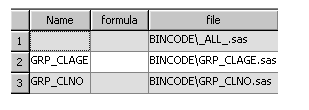Note that there is an individual sas file created for each of the new transformations. In addition, there is an entry for _ALL_.sas with no corresponding NAME or FORMULA value. This file should always be included when collecting the optimized score code and contains any code that may be replicated or used across all of the individual files. An example of code that may be found in this file is:

length _UFormat \$200;

drop _UFormat;

_UFormat='';

The _UFormat is a temporary variable used across the creation of several variables, but only needs to be defined and initialized once.

You can also go to the BINCODE subfolder found in your node folder and validate that 3 new .sas files exist. One for _ALL_, one for GRP_CLAGE and one for GRP_CLNO.

I next follow the steps for creating a Decision Tree model as was done in Example 1. I first view the variables passed into the Decision Tree node and verify that GRP_CLAGE and GRP_CLNO have both been created by the EMFLOWSCORECODE.sas file from my SAS Code node, and are available as splitting variables in the Decision Tree node. At this point, I open Interactive Training for the Decision Tree Node and force a split on the root node using my new variable GRP_CLAGE. I then save this change by closing interactive training. This will create a Decision Tree model in which only the GRP_CLAGE
variable is needed for scoring. I now run the Score Node and view the results of this node when the run completes.

You can see that the SAS Code displayed in the results of this node contains scoring code for ALL of the new transformations I created in the SAS Code node, in addition to the scoring code for the Decision Tree model (both GRP_CLAGE and GRP_CLNO are seen).

However, if I look to the Optimized SAS Code displayed in these results, I see that only GRP_CLAGE is included in the scoring code in the EMCODETOOL section of scoring code. Why? Because this is the only variable that was actually necessary for building the Decision Tree. There is no need for the optimized scoring code to create GRP_CLNO.

Have any other examples of simple or complex transformations? Find this tip useful or have questions? Please share your thoughts by commenting below.

Version history
Last update:
‎10-06-2015 01:45 PM
Updated by:Contributors
Article Labels
Article Tags